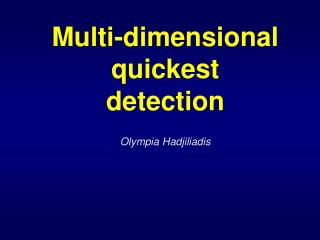DownloadDownload PresentationMulti-dimensional quickest detection

# Multi-dimensional quickest detection

Download Presentation## Multi-dimensional quickest detection

- - - - - - - - - - - - - - - - - - - - - - - - - - - E N D - - - - - - - - - - - - - - - - - - - - - - - - - - -
##### Presentation Transcript

2. Outline: Part I- One Shot Schemes • The multi-source quickest detection problem • The decentralized example &a synchronous communication through • CUSUM one shot schemes (& its optimality) • Multiple sources set-up of trade-off asap vs fa • Asymptotically there is no loss of information In the decentralized/centralized set-up (more mathematically….) • Asymptotic optimality of the N-CUSUM rule • Summary

3. We sequentially observe through independent sources i=1,…,N Information (if all at one location) with special attn to & The multi-source quickest detection OBJECTIVE: Detect the minimum of as soon as possible but controlling false alarms ASSUMPTION 1) The onset of signal can take place at distinct times :unknown constants

4. Lorden’s criterion (1971) subject to Optimality of the CUSUM Min-Max approach The Cumulative Sum (CUSUM) is optimal Shiryaev(1996), Beibel(1996)

5. T3 T2 T1 TN The decentralized system Each sensor Si is sequentially observing continuous observations S3 S2 … S1 SN Fusion center

6. subject to The CUSUM stopping rule is … and is optimal in CUSUM & its optimality The CUSUM statistic process is Asap Mean time to false alarm

7. Then and Multiple sources set-up subject to d Define The optimal stopping rule satisfies Proof: Let N=2 and consider S be s.t. Let U stop as S, when observing {ξt1} instead of {ξt2} & vice versa Consider T stops as S when Heads and as U when Tails while

8. Multiple sources set-up Natural candidate: N-CUSUM Hence the best N-CUSUM satisfies Which translates to (as γ→∞)

9. Asymptotic optimality as γ→∞ • If • If • If where

10. Asymptotic optimality (unequal strengths) N=2, µ1=1, µ2=1.2µ1

11. Asymptotic optimality(unequal strengths) N=2, µ1=1, µ2=1.5µ1

12. Outline: Part II-Coupled systems • The multi-source quickest detection problem • Models of general dependencies • Objective: Detect the first instance of a signal; Meaning: • Detect the min of N change points in Ito processes • Set-up the problem as a stochastic optimization w.r.t. a Kullback Leibler divergence • Asymptotic optimality of the N-CUSUM rule • Summary

13. We sequentially observe through independent sources i=1,…,N Information (if all at one location) with special attn to & The multi-source quickest detection OBJECTIVE: Detect the minimum of as soon as possible but controlling false alarms ASSUMPTION 1) The onset of signal can take place at distinct times 2) : unknown constants

14. Examples A system with AR behavior in each component and additive feedback from other sources Such a system with signals of different strengths in each sensor

15. CUSUM & its optimality N=1 The CUSUM statistic process is The CUSUM stopping rule is subject to is optimal in Asap Mean time to false alarm

16. ASSUMPTION are the same in law across all i Multiple sources set-up subject to d Define The optimal stopping rule satisfies

17. Since are the same across i… ALL ABOVE ARE EQUAL Multiple sources set-up Natural candidate: N-CUSUM Therefore…

18. To solve this problem we need… Take N=2. It is possible to show that satisfy

19. To solve this… • G is the probability that a particle placed at (x,y) will leave D after t.

20. NOTE: Asymptotic optimality as γ→∞ • If where

21. We sequentially observe through independent sources i=1,2 subject to Non-symmetric signals Suppose where

22. Multiple sources set-up • If as Natural candidate: 2-CUSUM In order to have an equalizer rule, or equivalently we need

23. We sequentially observe through independent sources i=1,2 subject to Non-symmetric signals Suppose where

24. Summary • Asymptotic optimality of the N-CUSUM rule in the case are the same across I • In the case of Brownian motions with const drift MESSAGE: If you want to detect the first instance of onset of a signal, let the sensors do the work! (Lose almost nothing in efficiency) • Extensions to the case different in law across i • What if the noises across sources are correlated.

25. Thanks to all collaborators • H. Vincent Poor • Tobias Schaefer • Hongzhong Zhang

26. “One-shot schemes for decentralized quickest change detection”, O. Hadjiliadis, H. Zhang and H. V. Poor, IEEE Transactions on Information Theory 55(7) 2009. • “Quickest Detection in coupled systems”, O. Hadjiliadis, T. Schaefer and H. V. Poor , Proceedings the 48th IEEE Conference on Decisions and Control, (2009) Submitted to the SIAM Journal on control and optimization (2010)

27. THE END